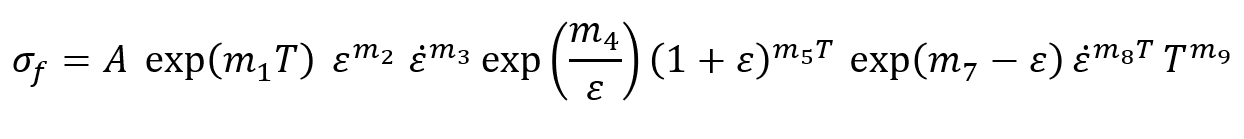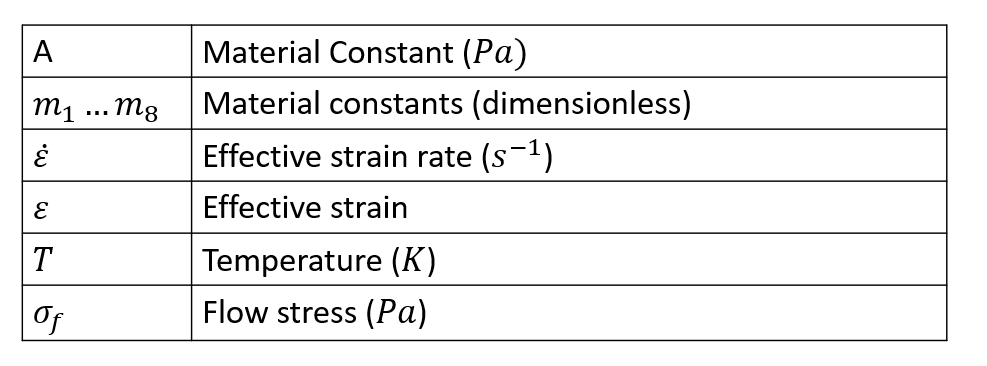# Hensel Spittel Model

Temperature, strain, and strain rate dependent Hensel Spittel model used in HyperXtrude is given by the following expression. It has a built-in temperature dependence in the equation and it is used all the time.Workpiece Aluminium { ConstitutiveModel = "HenselSpittel" Density = ρ SpecificHeat = Cp(T) Conductivity = K(T) CoeffOfThermalExpansion = βT VolumetricHeatSource = Qvol ReferenceTemperature = T LiquidusTemperature = Tl SolidusTemperature = Ts Coefficient_A = A Coefficient_m1 = m1 Coefficient_m2 = m2 Coefficient_m3 = m3 Coefficient_m4 = m4 Coefficient_m5 = m5 Coefficient_m7 = m7 Coefficient_m8 = m8 Coefficient_m9 = m9 MinStrainLimit = 0.5 MaxStrainLimit = 5.0 }